## Access NCERT Solutions for Class 6 Maths Chapter 14: Practical Geometry Exercise 14.5

### Access NCERT Solutions for Class 6 Maths Chapter 14: Practical Geometry Exercise 14.5

1. Drawof length 7.3 cm and find its axis of symmetry.

Solutions:

Following steps are followed to construct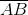of length 7.3 cm and to find its axis of symmetry

(1) Draw a line segmentof 7.3 cm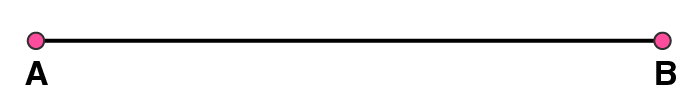(2) Take A as centre and draw a circle by using compasses. The radius of circle should be more than half the length of.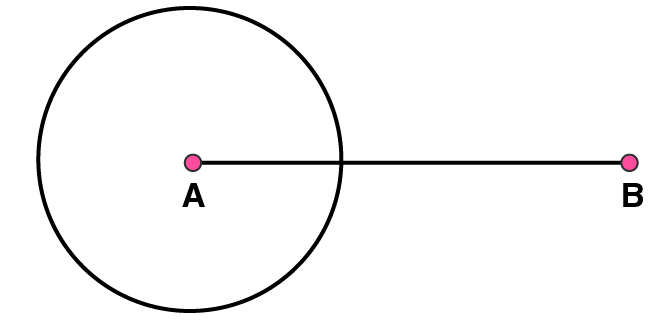(3) Now, take B as centre and draw another circle using compasses with the same radius as before. Let it cut the previous circle at points C and D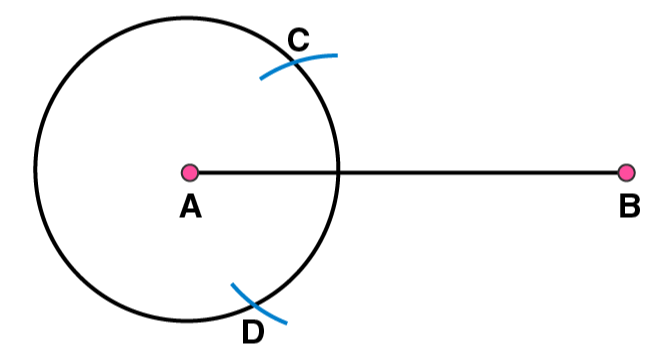(4) Join CD. Nowis the axis of symmetry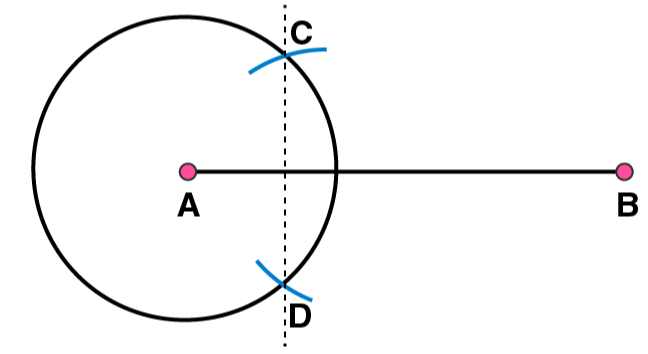2. Draw a line segment of length 9.5 cm and construct its perpendicular bisector.

Solutions:

Following steps are observed to construct a line segment of length 9.5 cm and to construct its perpendicular bisector

(1) Draw a line segment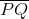of 9.5 cm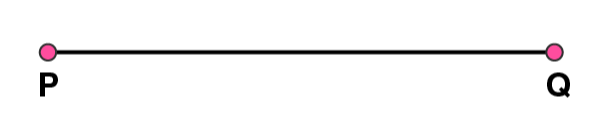(2) Take point P as centre and draw a circle by using compasses. The radius of circle should be more than half the length of(3) Taking the centre at point Q, again draw another circle using compasses with the same radius as before. Let it cut the previous circle at R and S respectively.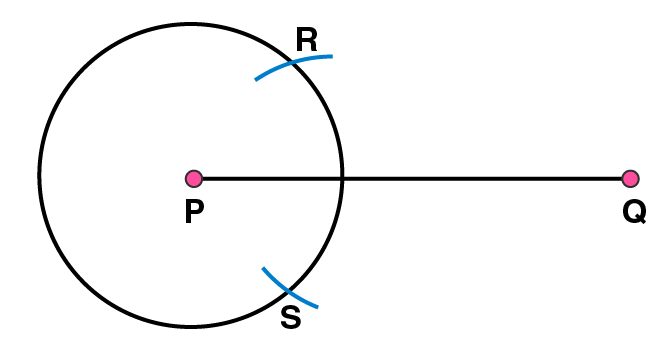(4) Join RS. Now,is the axis of symmetry i.e the perpendicular bisector of the line3. Draw the perpendicular bisector of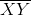whose length is 10.3 cm.

(a) Take any point P on the bisector drawn. Examine whether PX = PY.

(b) If M is the mid point of, what can you say about the lengths MX and XY?

Solutions:

(1) Draw a line segmentof 10.3 cm(2) Take point X as centre and draw a circle by using compasses. The radius of circle should be more than half the length of(3) Now taking Y as centre, draw another circle using compasses with the same radius as before. Let it cut at previous circle at points A and B(4) Join AB. Hereis the axis of symmetry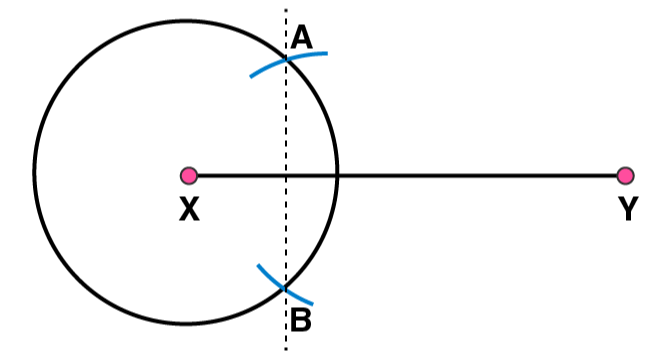(a) Take any point P on. We may observe that the measure of lengths of PX and PY are samebeing the axis of symmetry, any point lying onwill be at same distance from the both ends of(b) M is the midpoint of. Perpendicular bisectorwill be passing through point M. Hence length ofis double ofor 2MX = XY.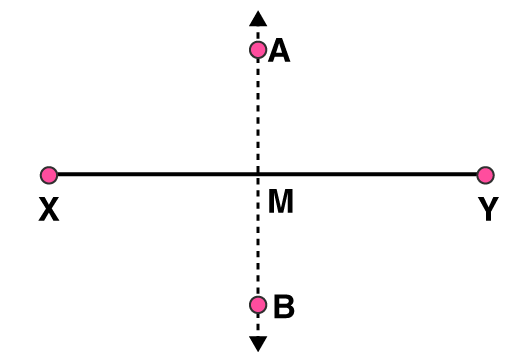4. Draw a line segment of length 12.8 cm. Using compasses, divide it into four equal parts. Verify by actual measurement.

Solutions:

(1) Draw a line segmentof 12.8 cm(2) By taking point X as centre and radius more than half of XY, draw a circle.(3) Again with same radius and centre as Y, draw two arcs to cut the circle at points A and B. Join AB which intersectsat point M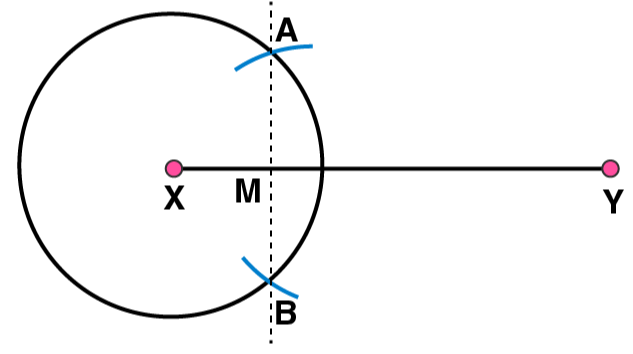(4) By taking X and Y as centres, draw two circles with radius more than half of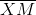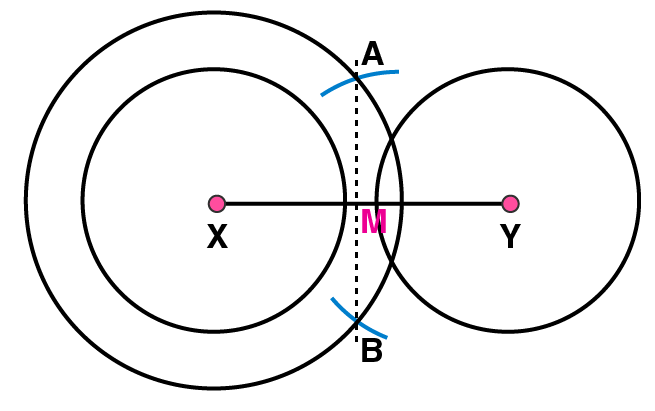(5) Taking M as centre and with same radius, draw two arcs to intersect these circles at P, Q and R, S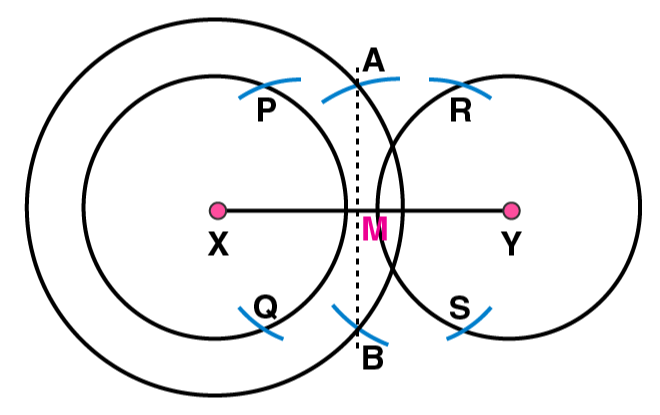(6) Join PQ, and RS. These are intersectingat points T and U.(7) The 4 equal parts ofare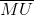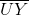BY measuring these line segments with the help of a ruler, we may observe that each is of 3.2 cm

5. Withof length 6.1 cm as diameter, draw a circle.

Solutions:

(1) Draw a line segmentof 6.1 cm(2) Take point P as centre and radius more than half of, draw a circle(3) Again with same radius and Q as centre, draw two arcs intersecting the circle at points R and S(4) Join RS which intersectsat T.(5) Taking the centre as T and radius TP, draw a circle which passes through Q. Now, this is the required circle.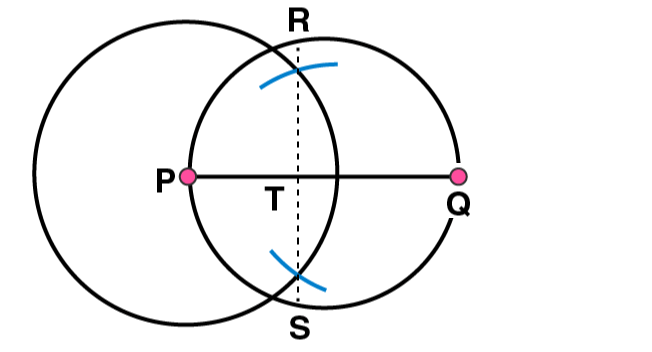6. Draw a circle with centre C and radius 3.4 cm. Draw any chord. Construct the perpendicular bisector ofand examine if it passes through C.

Solutions:

(1) Mark any point C on the sheet

(2) Adjust the compasses up to 3.4 cm and by putting the pointer of compasses at point C, turn compasses slowly to draw the circle. This is the required circle of 3.4 cm radius.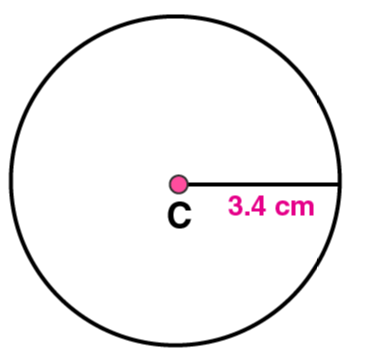(3) Mark any chordin the circle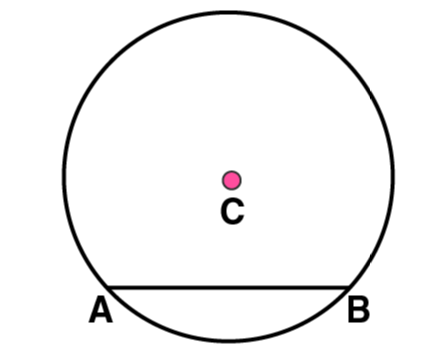(4) Now, taking A and B as centres, draw arcs on both sides of. Let these intersect each other at points D and E.(5) Join DE. Now DE is the perpendicular bisector of AB.If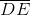is extended, it will pass through point C.

7. Repeat Question 6, ifhappens to be a diameter.

Solutions:

(1) Mark any point C on the sheet.

(2) Adjust the compasses up to 3.4 cm and by putting the pointer of compasses at point C, Turn the compasses slowly to draw the circle. This is the required circle of 3.4 cm(3) Now mark any diameterin the circle.(4) Now taking A and B as centres, draw arcs on both sides ofwith radius more than. Let these intersect each other at points D and E.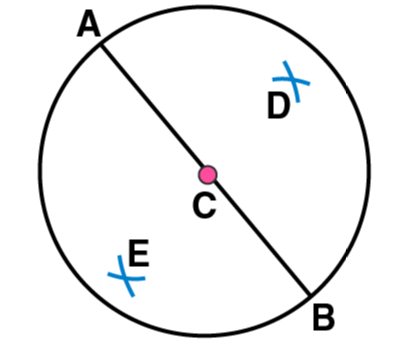(5) Join DE, which is perpendicular bisector of AB.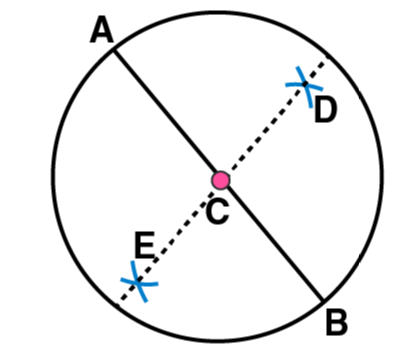Now, we may observe thatis passing through the centre C of the circle.

8. Draw a circle of radius 4 cm. Draw any two of its chords. Construct the perpendicular bisectors of these chords. Where do they meet?

Solutions:

(1) Mark any point O on the sheet. Now adjust the compasses up to 4 cm and by placing the pointer of compasses at point O, turn the compasses slowly to draw the circle. This is the required circle of 4 cm radius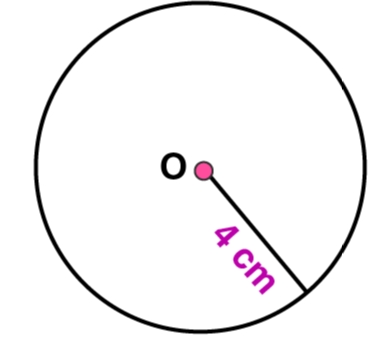(2) Take any two chordsand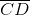in the circle(3) By taking A and B as centres and radius more than half of, draw arcs on both sides of AB. The arcs are intersecting each other at point E and F. Join EF which is perpendicular bisector of AB.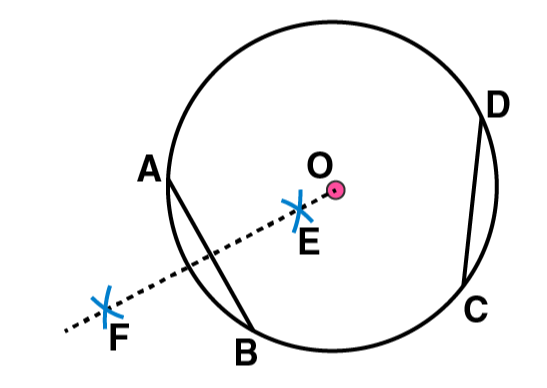(4) Again take C and D as centres and radius more than half of, draw arcs on both sides of CD such that they are intersecting each other at points G, H. Join GH which is perpendicular bisector of CDWe may observe that when EF and GH are extended they meet at the point O, which is the centre of circle

9. Draw any angle with vertex O. Take a point A on one of its arms and B on another such that OA = OB. Draw the perpendicular bisectors ofand. Let them meet at P. Is PA = PB?

Solutions:

(1) Draw any angle with vertex as O.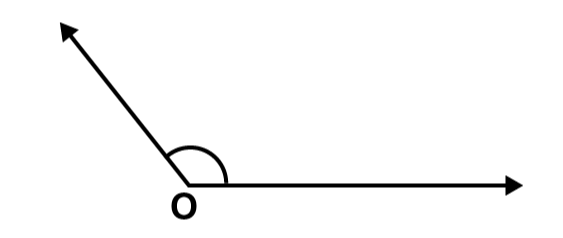(2) By taking O as centre and with convenient radius, draw arcs on both rays of this angle. Let these points are A and B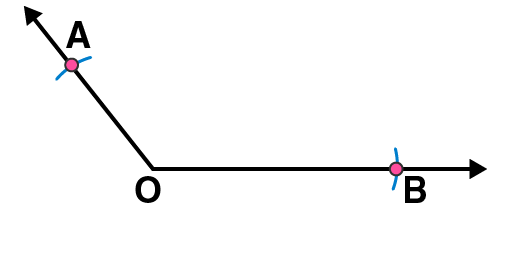(3) Now take O and A as centres and with radius more than half of OA, draw arcs on both sides of OA. Let these intersects at points C and D respectively. Join CD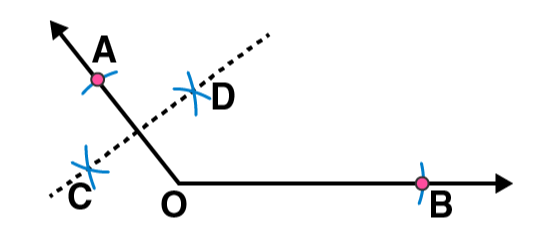(4) Similarly we may findwhich is perpendicular bisector of. These perpendicular bisectorsand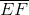intersects each other at point P. Now measure PA and PB. They are equal in length.